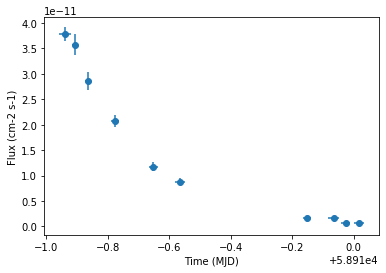This is a fixed-text formatted version of a Jupyter notebook

# Binned light curve simulation and fitting¶

## Context¶

Frequently, studies of variable sources (eg: decaying GRB light curves, AGN flares, etc) require time variable simulations. For most use cases, generating an event list is an overkill, and it suffices to use binned simulations using a temporal model.

Objective: Simulate and fit a time decaying light curve of a source with CTA using the CTA 1DC response

## Proposed approach:¶

We will simulate 10 spectral datasets within given time intervals (Good Time Intervals) following a given spectral (a power law) and temporal profile (an exponential decay, with a decay time of 6 hr ). These are then analysed using the light curve estimator to obtain flux points. Then, we re-fit the simulated datasets to reconstruct back the injected profiles.

In summary, necessary steps are:

• Choose observation parameters including a list of gammapy.data.GTI

• Define temporal and spectral models from :ref:model-gallery as per science case

• Perform the simulation (in 1D or 3D)

• Extract the light curve from the reduced dataset as shown in light curve notebook

• Optionaly, we show here how to fit the simulated datasets using a source model

## Setup¶

:

%matplotlib inline
import matplotlib.pyplot as plt
import numpy as np
import astropy.units as u
from astropy.coordinates import SkyCoord, Angle
from astropy.time import Time
from regions import CircleSkyRegion

import logging

log = logging.getLogger(__name__)


And some gammapy specific imports

:

from gammapy.data import Observation
from gammapy.datasets import SpectrumDataset, Datasets
from gammapy.modeling.models import (
PowerLawSpectralModel,
ExpDecayTemporalModel,
SkyModel,
)
from gammapy.maps import MapAxis
from gammapy.estimators import LightCurveEstimator
from gammapy.makers import SpectrumDatasetMaker
from gammapy.modeling import Fit


## Simulating a light curve¶

We will simulate 10 datasets using an PowerLawSpectralModel and a ExpDecayTemporalModel. The important thing to note here is how to attach a different GTI to each dataset.

:

# Loading IRFs
"\$GAMMAPY_DATA/cta-1dc/caldb/data/cta/1dc/bcf/South_z20_50h/irf_file.fits"
)

:

# Reconstructed and true energy axis
center = SkyCoord(0.0, 0.0, unit="deg", frame="galactic")
energy_axis = MapAxis.from_edges(
np.logspace(-0.5, 1.0, 10), unit="TeV", name="energy", interp="log"
)
energy_axis_true = MapAxis.from_edges(
np.logspace(-1.2, 2.0, 31), unit="TeV", name="energy", interp="log"
)


:

# Pointing position
pointing = SkyCoord(0.5, 0.5, unit="deg", frame="galactic")


Note that observations are usually conducted in Wobble mode, in which the source is not in the center of the camera. This allows to have a symmetrical sky position from which background can be estimated.

:

# Define the source model: A combination of spectral and temporal model

gti_t0 = Time("2020-03-01")
spectral_model = PowerLawSpectralModel(
index=3, amplitude="1e-11 cm-2 s-1 TeV-1", reference="1 TeV"
)
temporal_model = ExpDecayTemporalModel(t0="6 h", t_ref=gti_t0.mjd * u.d)
model_simu = SkyModel(
spectral_model=spectral_model,
temporal_model=temporal_model,
name="model-simu",
)

:

# Look at the model
model_simu.parameters.to_table()

:

Table length=5
namevalueunitminmaxfrozenerror
str9float64str14float64float64boolint64
index3.000e+00nannanFalse0.000e+00
amplitude1.000e-11cm-2 s-1 TeV-1nannanFalse0.000e+00
reference1.000e+00TeVnannanTrue0.000e+00
t02.500e-01dnannanFalse0.000e+00
t_ref5.891e+04dnannanTrue0.000e+00

Now, define the start and observation livetime wrt to the reference time, gti_t0

:

n_obs = 10
tstart = [1, 2, 3, 5, 8, 10, 20, 22, 23, 24] * u.h
lvtm = [55, 25, 26, 40, 40, 50, 40, 52, 43, 47] * u.min


Now perform the simulations

:

datasets = Datasets()

empty = SpectrumDataset.create(
e_reco=energy_axis.edges,
e_true=energy_axis_true.edges,
region=on_region,
name="empty",
)

for i in range(n_obs):
obs = Observation.create(
pointing=pointing,
livetime=lvtm[i],
tstart=tstart[i],
irfs=irfs,
reference_time=gti_t0,
)
empty_i = empty.copy(name=f"dataset_{i}")
maker = SpectrumDatasetMaker(selection=["aeff", "background", "edisp"])
dataset = maker.run(empty_i, obs)
dataset.models = model_simu
dataset.fake()
datasets.append(dataset)


The reduced datasets have been successfully simulated. Let’s take a quick look into our datasets.

:

datasets.info_table()

:

Table length=10
namelivetimen_onbackgroundexcesssignificancebackground_rategamma_rate
s1 / s1 / s
str9float64int64float64float64float64float64float64
stacked3299.99999999999979620.30377224384548775.696227756154565.492940017483620.0061526582557107530.2350594629564105
dataset_11500.03439.228987383566126333.7710126164338442.574750955469160.0061526582557107510.2225140084109559
dataset_21560.02879.598146878908771277.401853121091237.357701735706510.0061526582557107510.17782170071864822
dataset_32400.032414.766379813705804309.2336201862941637.1861511610167240.0061526582557107510.12884734174428925
dataset_42399.999999999996419114.766379813705779176.2336201862942525.008446122692540.006152658255710750.07343067507762271
dataset_53000.018218.457974767132253163.5420252328677622.4929689593655480.0061526582557107510.05451400841095592
dataset_62400.03814.76637981370580423.2336201862941965.0369120121051790.0061526582557107510.009680675077622582
dataset_73120.05119.19629375781754331.8037062421824576.0048018205659060.0061526582557107510.010193495590443096
dataset_82580.02615.87385829973373910.1261417002662612.32501787631360470.0061526582557107510.00392486112413421
dataset_92820.02917.3504962811043211.6495037188956822.5483575723723540.0061526582557107520.00413102968755166

## Extract the lightcurve¶

This section uses standard light curve estimation tools for a 1D extraction. Only a spectral model needs to be defined in this case. Since the estimator returns the integrated flux separately for each time bin, the temporal model need not be accounted for at this stage.

:

# Define the model:
spectral_model = PowerLawSpectralModel(
index=3, amplitude="1e-11 cm-2 s-1 TeV-1", reference="1 TeV"
)
model_fit = SkyModel(spectral_model=spectral_model, name="model-fit",)

:

# Attach model to each dataset
for dataset in datasets:
dataset.models = model_fit

:

%%time
lc_maker_1d = LightCurveEstimator(
energy_range=[energy_axis.edges, energy_axis.edges[-1]],
source="model-fit",
reoptimize=False,
)
lc_1d = lc_maker_1d.run(datasets)

CPU times: user 1.16 s, sys: 16.6 ms, total: 1.17 s
Wall time: 1.2 s

:

ax = lc_1d.plot(marker="o", label="3D")We have the reconstructed lightcurve at this point. Further standard analyis might involve modeling the temporal profiles with an analytical or theoretical model. You may do this using your favourite fitting package, one possible option being curve_fit inside scipy.optimize.

In the next section, we show how to simulatenously fit the all datasets using a given temporal model. This does a joint fitting across the different datasets, while simultaneously miniminsing across the temporal model parameters as well. We will fit the amplitude, spectral index and the decay time scale. Note that t_ref should be fixed by default for the ExpDecayTemporalModel.

For modelling and fitting more complex flares, you should attach the relevant model to each group of datasets. The paramters of a model in a given group of dataset will be tied. For more details on joint fitting in gammapy, see here.

## Fit the datasets¶

:

# Define the model:

spectral_model1 = PowerLawSpectralModel(
index=2.0, amplitude="1e-12 cm-2 s-1 TeV-1", reference="1 TeV"
)
temporal_model1 = ExpDecayTemporalModel(t0="10 h", t_ref=gti_t0.mjd * u.d)
model = SkyModel(
spectral_model=spectral_model1,
temporal_model=temporal_model1,
name="model-test",
)

:

model.parameters.to_table()

:

Table length=5
namevalueunitminmaxfrozenerror
str9float64str14float64float64boolint64
index2.000e+00nannanFalse0.000e+00
amplitude1.000e-12cm-2 s-1 TeV-1nannanFalse0.000e+00
reference1.000e+00TeVnannanTrue0.000e+00
t04.167e-01dnannanFalse0.000e+00
t_ref5.891e+04dnannanTrue0.000e+00
:

for dataset in datasets:
dataset.models = model

:

%%time
# Do a joint fit
fit = Fit(datasets)
result = fit.optimize()

CPU times: user 8.36 s, sys: 111 ms, total: 8.47 s
Wall time: 9.03 s

:

result.parameters.to_table()

:

Table length=5
namevalueunitminmaxfrozenerror
str9float64str14float64float64boolint64
index2.983e+00nannanFalse0.000e+00
amplitude9.907e-12cm-2 s-1 TeV-1nannanFalse0.000e+00
reference1.000e+00TeVnannanTrue0.000e+00
t02.497e-01dnannanFalse0.000e+00
t_ref5.891e+04dnannanTrue0.000e+00

We see that the fitted parameters match well with the simulated ones!

## Exercises¶

1. Re-do the analysis with MapDataset instead of SpectralDataset

2. Model the flare of PKS 2155-304 which you obtained using the light curve flare tutorial. Use a combination of a Gaussian and Exponential flare profiles, and fit using scipy.optimize.curve_fit

3. Do a joint fitting of the datasets.

[ ]: